# AP Physics 1 Multiple-Choice Practice Test 27

### Test Information10 questions18 minutes

1. A student is experimenting with a simple spring-block oscillator of spring constant k and amplitude A. The block attached to the spring has a mass of M. If the student places a small block of mass m on top of the original block, which of the following is true?

2. A flute supports standing waves with pressure nodes at each end. The lowest note a flute can play is 261.63 Hz. What is the approximate length of the flute? (speed of sound in air = 343 m/s)

3. You are standing on a railroad track as a train approaches at a constant velocity. Suddenly the engineer sees you, applies the brakes, and sounds the whistle. Which of the following describes the sound of the whistle while the train is slowing down, as you hear it?

4. Question refers to the following figure: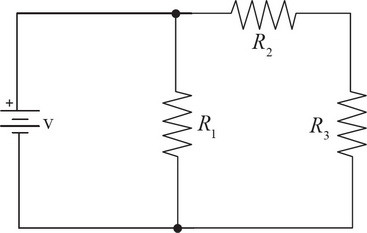Determine the total power dissipated through the circuit shown above in terms of V, R1, R2, and R3.

5. Question refers to the following figure:If V = 100 V, R1 = 50 Ω, R2 = 80 Ω, and R3 = 120 Ω, determine the voltage across R3.

6. Question refers to the following figure:If R1 were to burn out, the current coming out from the battery would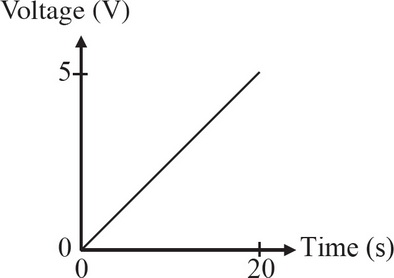7. A circuit consists of a 500 Ω resistor connected to a variable voltage source. The voltage is increased linearly from 0 V to 5 V over a period of 20 s, as shown in the graph above. Which of the following graphs corresponds to the power dissipated by the resistor as a function of time?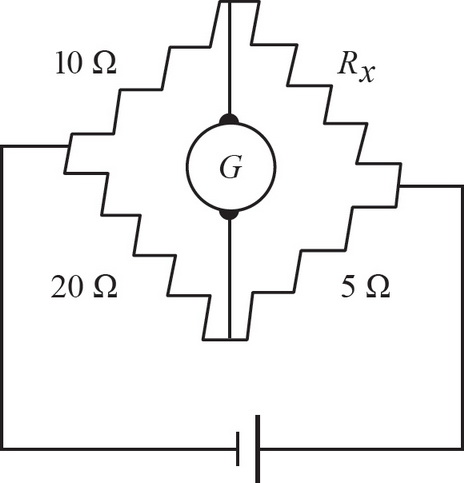8. A Wheatstone bridge (diagram above) is a configuration of resistors and a sensitive current meter, called a galvanometer, that is used to determine the resistance of an unknown resistor. In the Wheatstone bridge shown here, find the value of Rx such that the current through galvanometer G is zero.

9. What happens to the electric force between two point charges if the magnitude of both charges is doubled, and the distance between them is halved?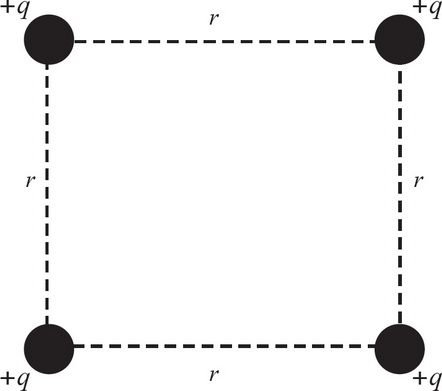10. In the figure above, four charges are arranged. If the magnitudes of all the charges q are all the same and the distance r between them is as shown above, what is the magnitude of the net force on the bottom right charge in terms of q, r, and k (where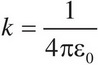)?